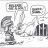G

#### Gildes

##### New member
Hi, I am trying to get an alert on my watchlist when a fractal has formed using this script, I know I might get a delay on the alert.
all ideas are welcome.

Thank you

Code:
``````# Bill Williams Fractal Template
# Coded By: Rigel May 2018

#Define "n" as the number of periods and keep a minimum value of 2 for error handling.
input n=2;

# Williams Fractals are a 5 point lagging indicator that will draw 2 candles behind.
# The purpose of the indicator is to plot points of trend reversals.
# Often these are paired with trailing stop indicators such as Parabolic SAR, Volatility Stop, and SuperTrend.

# Down pointing fractals occur over candles when:
#   High(n-2) < High(n)
#   High(n-1) < High(n)
#   High(n + 1) < High(n)
#   High(n + 2) < High(n)
#dnFractal = (high[n-2] < high[n]) and (high[n-1] < high[n]) and (high[n+1] < high[n]) and (high[n+2] < high[n])

def isupfractal = if low < low and low < low and low < low[-1] and low < low[-2] then low else double.nan;
# Up pointing fractals occur under candles when:
#   Low(n-2) > Low(n)
#   Low(n-1) > Low(n)
#   Low(n + 1) > Low(n)
#   Low(n + 2) > Low(n)
#upFractal = (low[n-2] > low[n]) and (low[n-1] > low[n]) and (low[n+1] > low[n]) and (low[n+2] > low[n])
def isdownfractal = if high > high and high > high and high > high[-1] and high > high[-2] then high else double.nan;
# Plot the fractals as shapes on the chart.

plot upfractal = if( isupfractal, isupfractal+ (1 * tickSize()) , double.nan);
upfractal.SetPaintingStrategy(paintingStrategy.ARROW_UP);
plot downfractal = if( isdownfractal, isdownfractal - (1 * tickSize()), double.nan);
downfractal.SetPaintingStrategy(paintingStrategy.ARROW_DOWN);``````

Last edited by a moderator:Indicators 7Indicators 16Tutorials 4